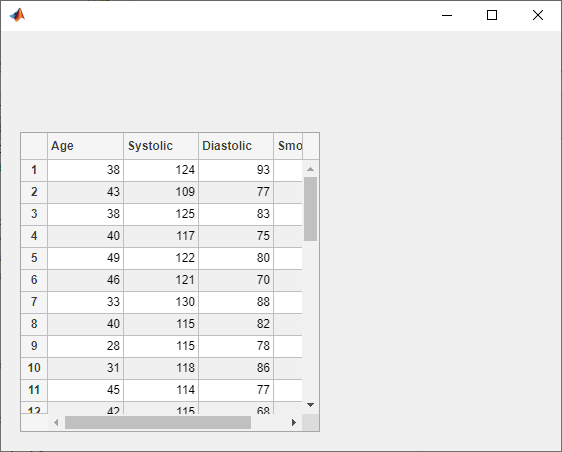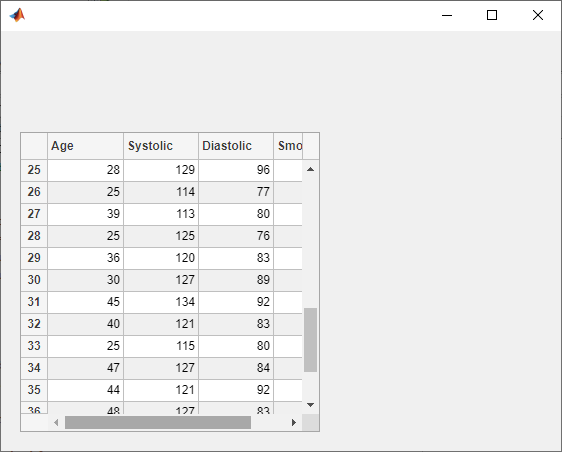uitable

说明

uit = uitable 在当前图窗中创建表用户界面组件，并返回 Table UI 组件对象。如果没有可用的图窗，MATLAB® 将调用 figure 函数创建一个图窗。

uit = uitable(Name,Value) 使用一个或多个名称-值对组参数指定表 UI 组件的属性值。

uit = uitable(parent) 在指定的父容器中创建表。父容器可以是使用 figureuifigure 函数创建的图窗，也可以是子容器（如面板）。uitable 的属性值略有不同，具体取决于该 App 是使用 figure 还是 uifigure 函数创建的。有关详细信息，请参阅名称-值对组参数

uit = uitable(parent,Name,Value) 指定父容器和一个或多个属性值。

示例

vars = {'Age','Systolic','Diastolic','Smoker'};
t = t(1:15,vars);

fig = uifigure;
uit = uitable(fig,'Data',t);vars = {'Year','MaxHeight','Validity'};
t = t(1:20,vars);
fig = uifigure;
uit = uitable(fig,'Data',t);uit.Data.Validity(1) = {'definite tsunami'};uit.Data.MaxHeight = uit.Data.MaxHeight*3.281;• 通过调用 readtable 函数创建一个 table 数组。

• 创建一个 UI 图窗。

• 创建一个可排序且可编辑的表 UI 组件以在图窗中显示。将 table 数组存储到组件的 Data 属性中。

• 创建一个气泡图来可视化海啸数据，其中气泡的坐标表示海啸的纬度和经度，气泡的大小表示海啸的最大高度。

• 指定一个 DisplayDataChangedFcn 回调，当 App 用户在表 UI 组件中对列进行排序或编辑单元格时，该回调使用 DisplayData 属性更新气泡图。

function tsunamisData
% Create table array
vars = {'Latitude','Longitude','MaxHeight'};
t = t(1:20,vars);

% Create UI figure
fig = uifigure;
fig.Position(3:4) = [722 360];

% Create table UI component
uit = uitable(fig);
uit.Data = t;
uit.ColumnSortable = true;
uit.ColumnEditable = [false false true];
uit.Position(3) = 290;
uit.DisplayDataChangedFcn = @updatePlot;

% Create bubble chart
ax = uiaxes(fig);
ax.Position(1) = 315;
ax.XLabel.String = 'Longitude';
ax.YLabel.String = 'Latitude';
x = t.Longitude;
y = t.Latitude;
sz = t.MaxHeight;
bubblechart(ax,x,y,sz)

% Update the bubble chart when table data changes
function updatePlot(src,event)
t = uit.DisplayData;
x = t.Longitude;
y = t.Latitude;
sz = t.MaxHeight;
bubblechart(ax,x,y,sz)
end

endvars = {'Year','Month','Day','Hour', ...
'MaxHeight','Cause','EarthquakeMagnitude'};
tdata = tdata(1:100,vars);

fig = uifigure('Position',[500 500 760 360]);
uit = uitable(fig);
uit.Position = [20 20 720 320];
uit.Data = tdata;
uit.RowName = 'numbered';

styleIndices = ismissing(tdata);
[row,col] = find(styleIndices);

s = uistyle('BackgroundColor',[1 0.6 0.6]);vars = {'Age','Systolic','Diastolic','Smoker'};
tdata = tdata(1:40,vars);

fig = uifigure;
uit = uitable(fig,'Data',tdata);
uit.RowName = 'numbered';scroll(uit,'row',25)f = figure;
uit = uitable(f,'Data',randi(100,10,3),'Position',[20 20 262 204]);f = figure;
uit = uitable(f);

d = {'Male',52,true;'Male',40,true;'Female',25,false};
uit.Data = d;
uit.Position = [20 20 258 78];uit.ColumnName = {'Gender','Age','Authorized'};
uit.ColumnEditable = true;输入参数

• 面板、选项卡和按钮组可以是任一类型的图窗中的容器。

• 网格布局是只能位于使用 uifigure 函数创建的图窗中的容器。

名称-值对组参数

• 有关对通过 uifigure 函数或 App 设计工具创建的 App 可用的属性列表，请参阅 Table 属性

• 有关对通过 figure 函数创建的 App 可用的属性列表，请参阅 Table 属性